# Solving Equations with Multiplication/Division

 In the previous lesson, we discussed that the goal in solving equations is to get the variable all by itself on one side of the equal sign. In order to do that, you need to move everything else to the other side of the equation. Also remember that while you are working with an equation, you have to keep it balanced. What that means is that you can multiply the equation by any number as long as you do it to both sides. In order to move a number that is multiplied or divided by the variable, you must do the inverse to both sides. That means, if the number is multiplied, you must divide it by both sides. If it's divided, you must multiply it by both side. Example: 1) 3x=10 Even if you can do this in your head, you need to learn the steps so that you can apply them to more complex problems. Our goal is to get the x by itself, so we first need to figure out what needs to move. The 3 is on the same side as the x, so that is what we need to move. It's being multiplied, so we are going to divide both sides by 3. The easiest way to do this is to make them fractions and put 3 as the denominator.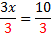Now we will work with each side. On the left hand side, the 3's will cancel out and disappear (3/3 = 1 and 1x = x).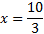We have the variable all by itself, so this is our answer. We could write it as a mixed number if we chose, but typically the improper fraction is acceptable. 3x = 10 We can check the answer by putting 10/3 back in the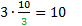true original equation in place of x. Final answer:This is true, so we know we have the right answer. 2)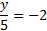Even if you can do this in your head, you need to learn the steps so that you can apply them to more complex problems. Our goal is to get the y by itself, so we first need to figure out what needs to move. The 5 is on the same side as the y, so that is what we need to move. It's being divide, so we are going to multiply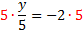both sides by 5. Now we will work with each side. On the left hand side, y = -10 the 5's will cancel out and disappear. On the right, -2(5) = -10 We have the variable all by itself, so this is our answer. We can check the answer by putting -10 back in the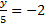original equation in place of y.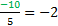true Final answer: y = -10 This is true, so we know we have the right answer. 3)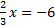The fraction 2/3 is on the same side as the x, so that is what we need to move. It's being multiplied, so we are going to divide both sides by 2/3. Remember that the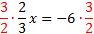way you divide a fraction is by flipping it and multiplying (called the reciprocal). So we will flip 2/3 upside down and multiply both sides by 3/2. x = -9 Now we will work with each side. On the left hand side, the 3/2 and 2/3 will cancel out and disappear. On the right,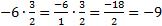We have the variable all by itself, so this is our answer.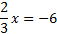We can check the answer by putting -9 back in the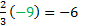original equation in place of x. Final answer: x = -9 This is true, so we know we have the right answer. Practice:Solve the equation and check your answer 1) 8x = -2 2)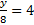3)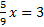4) -11 = 6a 5)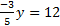Answers: 1)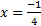2) y = 32 3)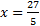4)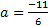5) y = -20

 Related Links: Math Algebra Equations Algebra Topics